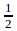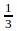Question Pool Pairs of Equations

# Pairs of Equations - SAMAGRA Question Pool & Answers | Class 9 English Medium

Kerala Syllabus SAMAGRA SCERT SAMAGRA Question Pool for Class 9 English Medium Maths Pairs of EquationsQn 1.

a) Three times a number added to another number gives 25.Taking the numbers as x and y form an equation ?

b) Instead of adding if the number is subtracted we get 17. Using this idea and form another equation ?

c) Find the numbers ?

3X + y = 25

3x – y =

Get Free Study Materials + 1 Week Free Trial of BrainsPrep Class 9 English Medium Tuition

Qn 2.

a)The sum of two numbers is 40. Taking the numbers as x and y form an equations ?

b)Their difference is 10. Using this idea form another equation?

c)Find the numbers ?

numbers x , y

x + y = 40 x- y = 10

numbers   25,15

Get Free Study Materials + 1 Week Free Trial of BrainsPrep Class 9 English Medium Tuition

Qn 3.

a)The difference between two number is 3.Take the numbers as x and y form an equation?

b)The difference of their squares is 39.Find the numbers?

let the numbers x,y

x-y =

Get Free Study Materials + 1 Week Free Trial of BrainsPrep Class 9 English Medium Tuition

Qn 4.

a)Write a fraction with numerator x and denominator y ?

b) “If its numerator is increased by 6 it became“If its denominator is increased by 7 it becameWrite the equations showing this relations ?

c) Find the fraction ?

Get Free Study Materials + 1 Week Free Trial of BrainsPrep Class 9 English Medium Tuition

Qn 5.

a)The hypotenuse of a right angled triangle is 15 cm. Taking the perpendicular sides as x , y write the relation connecting the sides ?

b)If the area is 30 cm² find the length of its perpendicular sides ?

Get Free Study Materials + 1 Week Free Trial of BrainsPrep Class 9 English Medium Tuition

Qn 6.

The length of a rectangle is 4 cm more than its breadth and its perimeter is 40 cm.

a) What is length + breadth ?

b) Find length and breadth by forming an equation ?

length = x+

Get Free Study Materials + 1 Week Free Trial of BrainsPrep Class 9 English Medium Tuition

Qn 7.

The price of a pen is Rs 5 more than the price of a pencil.

Total price of 3 pencil and 2 pen is Rs 45.

a) Take the price of pencil as x write the price of pen in terms of x ?

b) Find the price of pencil and pen by forming an equation ?

price of pencil = x

price of pen =

Get Free Study Materials + 1 Week Free Trial of BrainsPrep Class 9 English Medium Tuition

Qn 8.

a)Taking length as x and breadth as y write the area of the rectangle.

b)If the length is decreased by 1m and breadth is increased by 1m its area get increased by 3 m².Using this idea and form an equation.

c)If the length is increased by 1m and breadth is increased by 2m its area get increased by 19 m².Using this idea form another equation.

d)Find the length and breadth of the rectangle

length = x

area=

Get Free Study Materials + 1 Week Free Trial of BrainsPrep Class 9 English Medium Tuition

Qn 9.

Among two numbers one is 4 more than the other . Their sum is 40 .Take the small number as 'x'

i) Write large number in terms of x ?

ii) Find the numbers by forming an equation ?

small number– x

largenumber– x+4

sum = 40

x+( x+4) = 40

2x = 36

x = 18

numbers =

Get Free Study Materials + 1 Week Free Trial of BrainsPrep Class 9 English Medium Tuition

Qn 10.

a) The sum of digits of a two digit number is 9. Take digit in 10 th place as x and the digit in unit place as y form an equation using this idea ?

b) Write the number ?

c) If the digits are interchanged the number so obtained is 27 more than the actual number . Using this idea form an equation ?

d) Find the actual number ?

x+y =9

Get Free Study Materials + 1 Week Free Trial of BrainsPrep Class 9 English Medium Tuition

Qn 11.

a) Sabu's age is 7 times his son's age. Take son's age as x and write Sabu's age ?

b) Write their age after 8 years in terms of x ?

c) After 8 years Sabu's age is 3 times his son's age. Write this relation using an equation ?

d) Find their age now ?

son's age = x

sabu's age =

Get Free Study Materials + 1 Week Free Trial of BrainsPrep Class 9 English Medium Tuition

Qn 12.

a)Three times a number added to two times another number gives 33 . Taking the numbers as x and y form an equation using this idea ?

b)When three times the second number is subtracted from 4 times the first number got 10. Using this idea form another equation ?

c)Find the numbers ?

let numbers x and y

3x+2y =

Get Free Study Materials + 1 Week Free Trial of BrainsPrep Class 9 English Medium Tuition

Qn 13.

a)When a 500 rupee note was changed we got 20 rupee notes 50 rupee notes. Taking number of notes as x and y write an equation showing the total sum ?

b)If the total number of notes is 13 form another equation ?

c)Find the number of notes in each category ?

number of 20 rupee notes = x

number of 50 rupee notes = y

20x + 50y